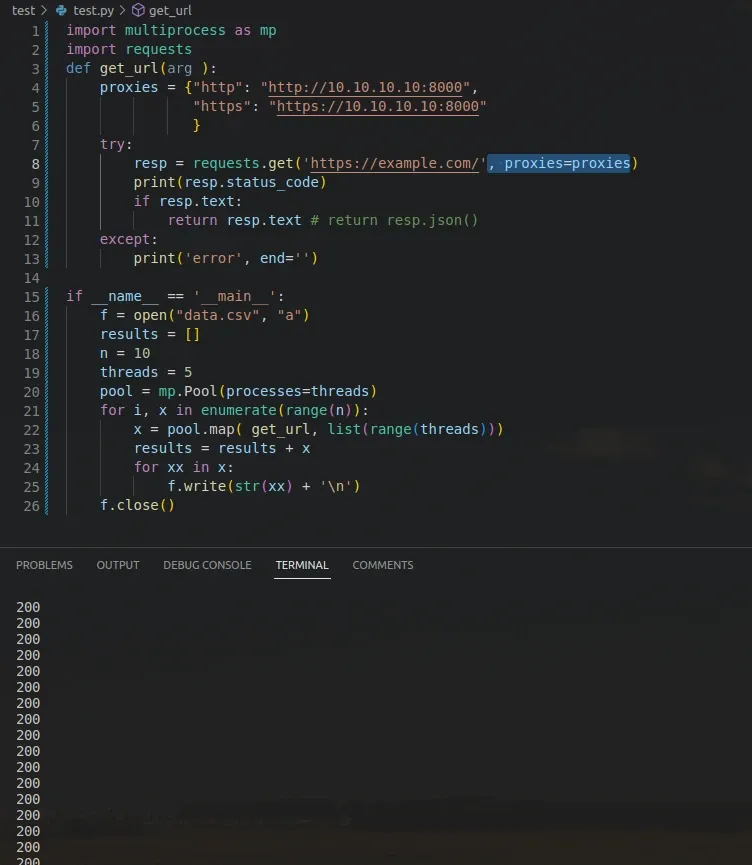To send multiple parallel HTTP requests in Python, we can use the `requests` library.

## send multiple HTTP GET requests

Let's start by example of sending multiple HTTP GET requests in Python:

``````import requests

urls = ['https://example.com/', 'https://httpbin.org/', 'https://example.com/test_page']

for url in urls:
response = requests.get(url)
print(response.status_code)
``````

We can find the status of each request below:

``````200
200
404
``````

## concurrent - multiple HTTP requests at once

To send multiple HTTP requests in parallel we can use Python libraries like:

• concurrent
• multiprocessing
• multiprocess
• asyncio

The easiest to use is the `concurrent` library.

Example of sending multiple GET requests with a `concurrent` library. We create a pool of worker threads or processes. In this example we have 3 concurrent requests - `max_workers=3`

``````import requests

urls = ['https://example.com/', 'https://httpbin.org/', 'https://example.com/test_page']

def get_url(url):
return requests.get(url)
print(list(pool.map(get_url,urls)))
``````

The result of the execution:

``````[<Response >, <Response >, <Response >]
``````

## asyncio & aiohttp - send multiple requests

If we want to send requests concurrently, you can use a library such as:

• `asyncio` in combination with
• `aiohttp`

Here's an example of how to use concurrent.futures to send multiple HTTP requests concurrently:

``````import aiohttp
import asyncio

urls = ['https://httpbin.org/ip', 'https://httpbin.org/get', 'https://httpbin.org/cookies'] * 10

async def get_url_data(url, session):
r = await session.request('GET', url=f'{url}')
data = await r.json()
return data

async def main(urls):
async with aiohttp.ClientSession() as session:
for url in urls:
return results

data = asyncio.run(main(urls))
for item in data:
print(item)
``````

Sending the multiple requests will result into simultaneous output:

``````{'origin': '123.123.123.123'}
{'args': {}, 'headers': {'Accept': '*/*', 'Accept-Encoding': 'gzip, deflate', 'Host': 'httpbin.org', 'User-Agent': 'Python/3.8 aiohttp/3.8.4', 'X-Amzn-Trace-Id': 'Root=1-63g7a39b-1ec5e2ed472cf9bf1874g45f'}, 'origin': '123.123.123.123', 'url': 'https://httpbin.org/get'}
``````

In the example above, we:

• create a list of URLs that we want to send requests to
• then create a two asynchronous method
• we extract JSON data from the result
• finally we print the results

## send multiple requests - proxy + file output

Finally let's see an example of sending multiple requests with:

• `multiprocess` library
• using proxy requests
• parsing JSON output
• saving the results into file:

The tests will run 10 times with 5 parallel processes. You need to use valid proxy and URL address in order to get correct results:

``````import multi process as mp
import requests
def get_url(arg ):
proxies = {"http": "http://10.10.10.10:8000",
"https": "https://10.10.10.10:8000"
}
try:
resp = requests.get('https://example.com/', proxies=proxies)
print(resp.status_code)
if resp.text:
return resp.text # return resp.json()
except:
print('error', end='')

if __name__ == '__main__':
f = open("data.csv", "a")
results = []
n = 10
for i, x in enumerate(range(n)):
results = results + x
for xx in x:
f.write(str(xx) + '\n')
f.close()
``````

result:

``````200
200
200
200
200
``````
Note:

Don't use these examples in Jupyter Notebook or JupyterLab. Parallel execution may not work as expected!

The result is visible on the image below:## summary

In this article we saw how to send multiple HTTP requests in Python with libraries like:

• concurrent
• multiprocessing
• multiprocess
• asyncio

We saw multiple examples with JSON data, proxy usage and file writing. We covered sending requests with libraries - `requests` and `aiohttp`

For more parallel examples in Python you can read: